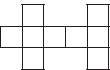# User ForumSubject :IMO    Class : Class 1

The given figure is made up of identical squares. The total area of the figure is 576 cm2. What is the perimeter of the figure?A 320 cm
B 160 cm
C 240 cm
D 200 cm

Class : Class 5

Class : Class 5

Class : Class 5

## Ans 4:

Class : Class 8
Number of squares in given figure = 9 Area of 9 squares = 576 cm 2 Area of 1 square = 576 Ã· 9 = 64 cm2 = 8 cm Ã 8 cm â´ Side of 1 square = 8 cm â´ Perimeter of the given figure = 20 Ã 8 cm = 160 cmSo the correct answer is B

Class : Class 5

Class : Class 6

Class : Class 5

## Ans 8:

Class : Class 6
How did 20 came? Please explain.